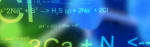All About Science# Third Law of Thermodynamics

QUESTION: What is the third law of thermodynamics?

The Third Law of Thermodynamics is the lesser known of the three major thermodynamic laws. Together, these laws help form the foundations of modern science. The laws of thermodynamics are absolute physical laws - everything in the observable universe is subject to them. Like time or gravity, nothing in the universe is exempt from these laws. In its simplest form, the Third Law of Thermodynamics relates the entropy (randomness) of matter to its absolute temperature.

The Third Law of Thermodynamics refers to a state known as "absolute zero." This is the bottom point on the Kelvin temperature scale. The Kelvin scale is absolute, meaning 0° Kelvin is mathematically the lowest possible temperature in the universe. This corresponds to about -273.15° Celsius, or -459.7 Fahrenheit.

In actuality, no object or system can have a temperature of zero Kelvin, because of the Second Law of Thermodynamics. The Second Law, in part, implies that heat can never spontaneously move from a colder body to a hotter body. So, as a system approaches absolute zero, it will eventually have to draw energy from whatever systems are nearby. If it draws energy, it can never obtain absolute zero. So, this state is not physically possible, but is a mathematical limit of the universe.

In its shortest form, the Third Law of Thermodynamics says: "The entropy of a pure perfect crystal is zero (0) at zero Kelvin (0° K)." Entropy is a property of matter and energy discussed by the Second Law of Thermodynamics. The Third Law of Thermodynamics means that as the temperature of a system approaches absolute zero, its entropy approaches a constant (for pure perfect crystals, this constant is zero). A pure perfect crystal is one in which every molecule is identical, and the molecular alignment is perfectly even throughout the substance. For non-pure crystals, or those with less-than perfect alignment, there will be some energy associated with the imperfections, so the entropy cannot become zero.

The Third Law of Thermodynamics can be visualized by thinking about water. Water in gas form has molecules that can move around very freely. Water vapor has very high entropy (randomness). As the gas cools, it becomes liquid. The liquid water molecules can still move around, but not as freely. They have lost some entropy. When the water cools further, it becomes solid ice. The solid water molecules can no longer move freely, but can only vibrate within the ice crystals. The entropy is now very low. As the water is cooled more, closer and closer to absolute zero, the vibration of the molecules diminishes. If the solid water reached absolute zero, all molecular motion would stop completely. At this point, the water would have no entropy (randomness) at all.

Most of the direct use of the Third Law of Thermodynamics occurs in ultra-low temperature chemistry and physics. The applications of this law have been used to predict the response of various materials to temperature changes. These relationships have become core to many science disciplines, even though the Third Law of Thermodynamics is not used directly nearly as much as the other two.

Study of the Third Law of Thermodynamics mainly supports the implications of the first two laws. However, some questions do arise. The Third Law of Thermodynamics demonstrates another detectable, absolute, all-encompassing, important natural law. The Laws of Thermodynamics demonstrate very ordered rules of energy interactions, which the entire universe must obey. Why is the Third Law of Thermodynamics true? Why are there no exceptions in the entire universe? This law cannot be an accident, since none of the thermodynamic laws are random. Organization implies an Organizer. Design implies a Designer. If the laws are not random, then who created them?